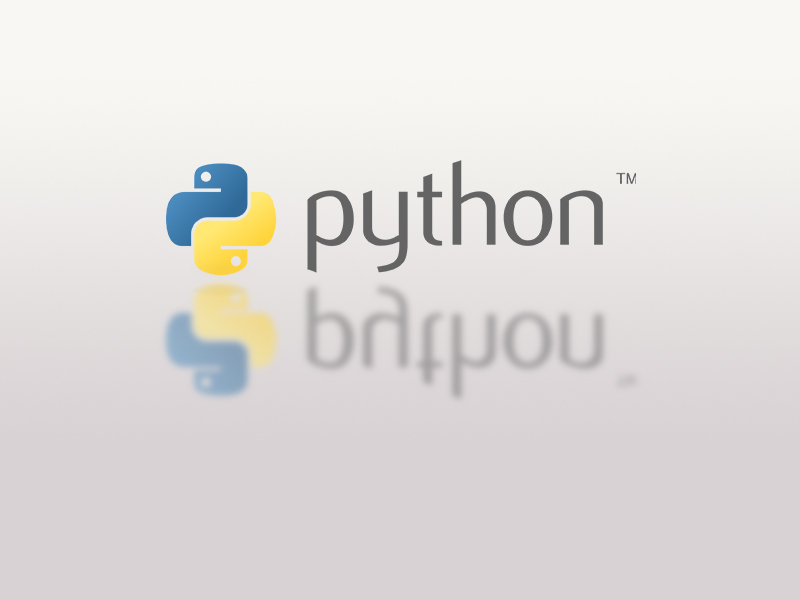# Python#### Lists in Python

You can find the python codes used in tutorial https://rajith681.wordpress.com/2016/06/18/python-3-lists below. List Creating List1=[] List2=[1,2,3,4,5] List3=['a','b','c'] List4=[1,'abc'] Accessing List Items List1=[] ...#### File Handling

Opening a file thefile=open("sample.txt","r") Here we are creating a file object name “thefile” and assigning the data in the “sample.txt” ...#### Insertion Sort Using Python

Read about Insertion Sort at http://interactivepython.org/runestone/static/pythonds/SortSearch/TheInsertionSort.html def insertionSort(alist): #defining the function for index in range(1,len(alist)): #run through the list form element ...#### Calculating Factorial Using Recursion

def fact(num): if num==1: return 1 else: return num*fact(num-1) print(fact(int(input("Please enter the number : ")))) ...#### Implementing a Queue in Python Using Lists

class Queue: def __init__(self): self.items = [] #create a new lsit with no items to use as the queue def ...#### Converting Decimal Numbers to Binary Numbers Using Stacks

This code will take an integer decimal number and convert it to a binary number. Here stack is used to ...#### Using Stacks to Reverse Four Letters

This code can be used to add four letters to a stack and get them in reverse order class Stack: ...#### Implementing Stacks in Python

What is a Stack? A stack is a collection, meaning that it is a data structure that contains multiple elements ...